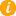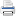Math K-Plus

TestQuizQuiz Results• Option 2. Solve a calculator generated math problem. First press the NEW PROBLEM button. Next enter your answer, in to the calculator. Lastly, press the ADD button and then compare your answer with the calculator's.

Field Name Subtract Values
Minuend: You can enter up to 2 digits
Subtrahend: You can enter up to 2 digitsAfter learning Third Grade Subtraction Calculator, you can move to the next step in subtraction math: Fourth Grade Subtraction Calculator.

+48
-  12
—————

48
-  12
—————

48
-  12
—————
6

48
-  12
—————
36

48
-  12
—————
+36